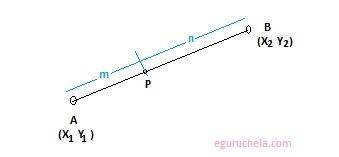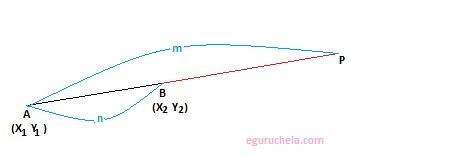# Coordinates of Points Externally/Internally Calculator

Please enter the values for point A and B (coordinates) and a specified ratio m and n

 Points X Y A: B:

 Ratio m n Value
 Externally Internally

Coordinates of Point:

Calculator find the coordinates of point p which divides the line joining two Entered points A and B internally or externally, in a specified ratio m and n. Coordinates of point is a set of values that is used to determine the position of a point in a two dimensional plane.

### Internally divided line segment:

The given coordinates forms a line AB where point P(xp, yp) lies outside of the line segment AB. It can divide the AB externally as showing below:#### Formula (Internally division of a line segment)

$$( \frac {mx_{\,2} + nx_{\,1} }{m + n},\frac {my_{\,2} + ny_{\,1} }{m + n} )$$

### Externally divided line segment:

The given coordinates forms a line AB where point P(xp, yp) lies on the line segment AB joining Point P. It is showing bellow:#### Formula (Externally division of a line segment)

$$( \frac {mx_{\,2} - nx_{\,1} }{m - n},\frac {my_{\,2} - ny_{\,1} }{m - n} )$$